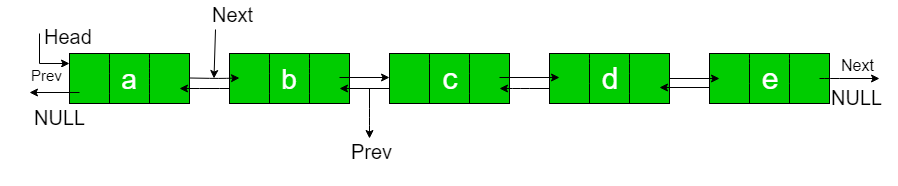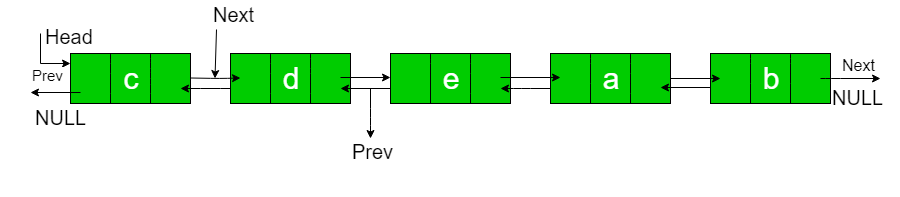Related Articles

# Rotate Doubly linked list by N nodes

• Difficulty Level : Easy
• Last Updated : 06 Oct, 2021

Given a doubly-linked list, rotate the linked list counter-clockwise by N nodes. Here N is a given positive integer and is smaller than the count of nodes in linked list.Attention reader! Don’t stop learning now. Get hold of all the important DSA concepts with the DSA Self Paced Course at a student-friendly price and become industry ready.  To complete your preparation from learning a language to DS Algo and many more,  please refer Complete Interview Preparation Course.

In case you wish to attend live classes with experts, please refer DSA Live Classes for Working Professionals and Competitive Programming Live for Students.

N = 2
Rotated List:Examples:

```Input : a  b  c  d  e   N = 2
Output : c  d  e  a  b

Input : a  b  c  d  e  f  g  h   N = 4
Output : e  f  g  h  a  b  c  d ```

1. To rotate the Doubly linked list, first, we need to traverse through the linked list and find the address of the last node.

2. Then make it a circular linked list.

3. Then move the head as well as a temp by n nodes.

4. Then make the linked list as un-circular.

Solution 1:

## C++

 `#include``using` `namespace` `std;` `class` `Node``{``    ``public``:``        ``char` `data;``        ``Node* next;``        ``Node* pre;``    ``Node(``int` `data)``    ``{``        ``this``->data=data;``        ``pre=NULL;``        ``next=NULL;``    ``}``};` `void` `insertAtHead(Node* &head, ``int` `data)``{``    ``Node* n = ``new` `Node(data);``    ``if``(head==NULL)``    ``{``        ``head=n;``        ``return``;``    ``}``    ``n->next=head;``    ``head->pre=n;``    ``head=n;``    ``return``;``}``void` `insertAtTail(Node* &head, ``int` `data)``{``    ``if``(head==NULL)``    ``{``        ``insertAtHead(head,data);``        ``return``;``    ``}``    ``Node* temp=head;``    ``while``(temp->next!=NULL)``    ``{``        ``temp=temp->next;``    ``}``    ``Node* n=``new` `Node(data);``    ``temp->next=n;``    ``n->pre=temp;``    ``return``;``}``void` `display(Node* head)``{``    ``while``(head!=NULL)``    ``{``        ``cout << head->data << ``"-->"``;``        ``head=head->next;``    ``}``    ``cout << ``"NULL\n"``;``}` `void` `rotateByN(Node* &head, ``int` `pos)``{``    ``// return without any changes if position is 0.``    ``if``(pos==0) ``return``;` `    ``// Finding last node.``    ``Node* temp=head;``    ``while``(temp->next!=NULL)``    ``{``        ``temp=temp->next;``    ``}``    ``// making the list circular.``    ``temp->next=head;``    ``head->pre=temp;` `    ``// move head and temp by the given position.``    ``int` `count=1;``    ``while``(count<=pos)``    ``{``        ``head=head->next;``        ``temp=temp->next;``        ``count++;``    ``}` `    ``// now again make list un-circular.``    ``temp->next=NULL;``    ``head->pre=NULL;``}``int` `main()``{``    ``Node* head=NULL;``    ``insertAtTail(head,``'a'``);``    ``insertAtTail(head,``'b'``);``    ``insertAtTail(head,``'c'``);``    ``insertAtTail(head,``'d'``);``    ``insertAtTail(head,``'e'``);` `    ``int` `n=2;``    ``cout << ``"\nBefore Rotation : \n"``;``    ``display(head);``    ``rotateByN(head,n);``    ``cout << ``"\nAfter Rotation : \n"``;``    ``display(head);``    ``cout << ``"\n\n"``;` `    ``return` `0;``}`

## Java

 `// Java program to rotate a Doubly linked``// list counter clock wise by N times``class` `GfG {` `/* Link list node */``static` `class` `Node``{``    ``char` `data;``    ``Node prev;``    ``Node next;``}``static` `Node head = ``null``;` `// This function rotates a doubly linked``// list counter-clockwise and updates the``// head. The function assumes that N is``// smallerthan size of linked list. It``// doesn't modify the list if N is greater``// than or equal to size``static` `void` `rotate( ``int` `N)``{``    ``if` `(N == ``0``)``        ``return``;` `    ``// Let us understand the below code``    ``// for example N = 2 and``    ``// list = a <-> b <-> c <-> d <-> e.``    ``Node current = head;` `    ``// current will either point to Nth``    ``// or NULL after this loop. Current``    ``// will point to node 'b' in the``    ``// above example``    ``int` `count = ``1``;``    ``while` `(count < N && current != ``null``)``    ``{``        ``current = current.next;``        ``count++;``    ``}` `    ``// If current is NULL, N is greater``    ``// than or equal to count of nodes``    ``// in linked list. Don't change the``    ``// list in this case``    ``if` `(current == ``null``)``        ``return``;` `    ``// current points to Nth node. Store``    ``// it in a variable. NthNode points to``    ``// node 'b' in the above example``    ``Node NthNode = current;` `    ``// current will point to last node``    ``// after this loop current will point``    ``// to node 'e' in the above example``    ``while` `(current.next != ``null``)``        ``current = current.next;` `    ``// Change next of last node to previous``    ``// head. Next of 'e' is now changed to``    ``// node 'a'``    ``current.next = head;` `    ``// Change prev of Head node to current``    ``// Prev of 'a' is now changed to node 'e'``    ``(head).prev = current;` `    ``// Change head to (N+1)th node``    ``// head is now changed to node 'c'``    ``head = NthNode.next;` `    ``// Change prev of New Head node to NULL``    ``// Because Prev of Head Node in Doubly``    ``// linked list is NULL``    ``(head).prev = ``null``;` `    ``// change next of Nth node to NULL``    ``// next of 'b' is now NULL``    ``NthNode.next = ``null``;``}` `// Function to insert a node at the``// beginning of the Doubly Linked List``static` `void` `push(``char` `new_data)``{``    ``Node new_node = ``new` `Node();``    ``new_node.data = new_data;``    ``new_node.prev = ``null``;``    ``new_node.next = (head);``    ``if` `((head) != ``null``)``        ``(head).prev = new_node;``head = new_node;``}` `/* Function to print linked list */``static` `void` `printList(Node node)``{``    ``while` `(node != ``null` `&& node.next != ``null``)``    ``{``        ``System.out.print(node.data + ``" "``);``        ``node = node.next;``    ``}``    ``if``(node != ``null``)``    ``System.out.print(node.data);``}` `// Driver's Code``public` `static` `void` `main(String[] args)``{``    ``/* Start with the empty list */``    ``// Node head = null;` `    ``/* Let us create the doubly``    ``linked list a<->b<->c<->d<->e */``    ``push( ``'e'``);``    ``push( ``'d'``);``    ``push(``'c'``);``    ``push(``'b'``);``    ``push( ``'a'``);` `    ``int` `N = ``2``;` `    ``System.out.println(``"Given linked list "``);``    ``printList(head);``    ``rotate( N);``    ``System.out.println();``    ``System.out.println(``"Rotated Linked list "``);``    ``printList(head);``}``}` `// This code is contributed by Prerna Saini`

## Python3

 `# Node of a doubly linked list``class` `Node:``    ``def` `__init__(``self``, ``next` `=` `None``,``                       ``prev ``=` `None``, data ``=` `None``):``        ``self``.``next` `=` `next` `# reference to next node in DLL``        ``self``.prev ``=` `prev ``# reference to previous node in DLL``        ``self``.data ``=` `data` `def` `push(head, new_data):` `    ``new_node ``=` `Node(data ``=` `new_data)` `    ``new_node.``next` `=` `head``    ``new_node.prev ``=` `None` `    ``if` `head ``is` `not` `None``:``        ``head.prev ``=` `new_node` `    ``head ``=` `new_node``    ``return` `head` `def` `printList(head):` `    ``node ``=` `head` `    ``print``(``"Given linked list"``)``    ``while``(node ``is` `not` `None``):``        ``print``(node.data, end ``=` `" "``),``        ``last ``=` `node``        ``node ``=` `node.``next``    ` `def` `rotate(start, N):``    ``if` `N ``=``=` `0` `:``        ``return` `    ``# Let us understand the below code``    ``# for example N = 2 and``    ``# list = a <-> b <-> c <-> d <-> e.``    ``current ``=` `start` `    ``# current will either point to Nth``    ``# or None after this loop. Current``    ``# will point to node 'b' in the``    ``# above example``    ``count ``=` `1``    ``while` `count < N ``and` `current !``=` `None` `:``        ``current ``=` `current.``next``        ``count ``+``=` `1` `    ``# If current is None, N is greater``    ``# than or equal to count of nodes``    ``# in linked list. Don't change the``    ``# list in this case``    ``if` `current ``=``=` `None` `:``        ``return` `    ``# current points to Nth node. Store``    ``# it in a variable. NthNode points to``    ``# node 'b' in the above example``    ``NthNode ``=` `current` `    ``# current will point to last node``    ``# after this loop current will point``    ``# to node 'e' in the above example``    ``while` `current.``next` `!``=` `None` `:``        ``current ``=` `current.``next` `    ``# Change next of last node to previous``    ``# head. Next of 'e' is now changed to``    ``# node 'a'``    ``current.``next` `=` `start` `    ``# Change prev of Head node to current``    ``# Prev of 'a' is now changed to node 'e'``    ``start.prev ``=` `current` `    ``# Change head to (N+1)th node``    ``# head is now changed to node 'c'``    ``start ``=` `NthNode.``next` `    ``# Change prev of New Head node to None``    ``# Because Prev of Head Node in Doubly``    ``# linked list is None``    ``start.prev ``=` `None` `    ``# change next of Nth node to None``    ``# next of 'b' is now None``    ``NthNode.``next` `=` `None` `    ``return` `start` `# Driver Code``if` `__name__ ``=``=` `"__main__"``:``    ``head ``=` `None` `    ``head ``=` `push(head, ``'e'``)``    ``head ``=` `push(head, ``'d'``)``    ``head ``=` `push(head, ``'c'``)``    ``head ``=` `push(head, ``'b'``)``    ``head ``=` `push(head, ``'a'``)` `    ``printList(head)``    ``print``(``"\n"``)``    ` `    ``N ``=` `2``    ``head ``=` `rotate(head, N)` `    ``printList(head)` `# This code is contributed by vinayak sharma`

## C#

 `// C# program to rotate a Doubly linked``// list counter clock wise by N times``using` `System;` `class` `GfG``{` `/* Link list node */``public` `class` `Node``{``    ``public` `char` `data;``    ``public` `Node prev;``    ``public` `Node next;``}``static` `Node head = ``null``;` `// This function rotates a doubly linked``// list counter-clockwise and updates the``// head. The function assumes that N is``// smallerthan size of linked list. It``// doesn't modify the list if N is greater``// than or equal to size``static` `void` `rotate( ``int` `N)``{``    ``if` `(N == 0)``        ``return``;` `    ``// Let us understand the below code``    ``// for example N = 2 and``    ``// list = a <-> b <-> c <-> d <-> e.``    ``Node current = head;` `    ``// current will either point to Nth``    ``// or NULL after this loop. Current``    ``// will point to node 'b' in the``    ``// above example``    ``int` `count = 1;``    ``while` `(count < N && current != ``null``)``    ``{``        ``current = current.next;``        ``count++;``    ``}` `    ``// If current is NULL, N is greater``    ``// than or equal to count of nodes``    ``// in linked list. Don't change the``    ``// list in this case``    ``if` `(current == ``null``)``        ``return``;` `    ``// current points to Nth node. Store``    ``// it in a variable. NthNode points to``    ``// node 'b' in the above example``    ``Node NthNode = current;` `    ``// current will point to last node``    ``// after this loop current will point``    ``// to node 'e' in the above example``    ``while` `(current.next != ``null``)``        ``current = current.next;` `    ``// Change next of last node to previous``    ``// head. Next of 'e' is now changed to``    ``// node 'a'``    ``current.next = head;` `    ``// Change prev of Head node to current``    ``// Prev of 'a' is now changed to node 'e'``    ``(head).prev = current;` `    ``// Change head to (N+1)th node``    ``// head is now changed to node 'c'``    ``head = NthNode.next;` `    ``// Change prev of New Head node to NULL``    ``// Because Prev of Head Node in Doubly``    ``// linked list is NULL``    ``(head).prev = ``null``;` `    ``// change next of Nth node to NULL``    ``// next of 'b' is now NULL``    ``NthNode.next = ``null``;``}` `// Function to insert a node at the``// beginning of the Doubly Linked List``static` `void` `push(``char` `new_data)``{``    ``Node new_node = ``new` `Node();``    ``new_node.data = new_data;``    ``new_node.prev = ``null``;``    ``new_node.next = (head);``    ``if` `((head) != ``null``)``        ``(head).prev = new_node;``    ``head = new_node;``}` `/* Function to print linked list */``static` `void` `printList(Node node)``{``    ``while` `(node != ``null` `&& node.next != ``null``)``    ``{``        ``Console.Write(node.data + ``" "``);``        ``node = node.next;``    ``}``    ``if``(node != ``null``)``    ``Console.Write(node.data);``}` `// Driver Code``public` `static` `void` `Main(String []args)``{``    ``/* Start with the empty list */``    ``// Node head = null;` `    ``/* Let us create the doubly``    ``linked list a<->b<->c<->d<->e */``    ``push( ``'e'``);``    ``push( ``'d'``);``    ``push( ``'c'``);``    ``push( ``'b'``);``    ``push( ``'a'``);` `    ``int` `N = 2;` `    ``Console.WriteLine(``"Given linked list "``);``    ``printList(head);``    ``rotate( N);``    ``Console.WriteLine();``    ``Console.WriteLine(``"Rotated Linked list "``);``    ``printList(head);``}``}` `// This code is contributed by Arnab Kundu`

## Javascript

 ``
```Output:
Before Rotation :
a-->b-->c-->d-->e-->NULL
After Rotation :
c-->d-->e-->a-->b-->NULL```

Time Complexity: O(N)

Space Complexity: O(1)

Solution 2:

## C++

 `#include``using` `namespace` `std;` `class` `Node``{``    ``public``:``        ``char` `data;``        ``Node* next;``        ``Node* pre;``    ``Node(``int` `data)``    ``{``        ``this``->data=data;``        ``pre=NULL;``        ``next=NULL;``    ``}``};` `void` `insertAtHead(Node* &head, ``int` `data)``{``    ``Node* n = ``new` `Node(data);``    ``if``(head==NULL)``    ``{``        ``head=n;``        ``return``;``    ``}``    ``n->next=head;``    ``head->pre=n;``    ``head=n;``    ``return``;``}``void` `insertAtTail(Node* &head, ``int` `data)``{``    ``if``(head==NULL)``    ``{``        ``insertAtHead(head,data);``        ``return``;``    ``}``    ``Node* temp=head;``    ``while``(temp->next!=NULL)``    ``{``        ``temp=temp->next;``    ``}``    ``Node* n=``new` `Node(data);``    ``temp->next=n;``    ``n->pre=temp;``    ``return``;``}``void` `display(Node* head)``{``    ``while``(head!=NULL)``    ``{``        ``cout << head->data << ``"-->"``;``        ``head=head->next;``    ``}``    ``cout << ``"NULL\n"``;``}` `void` `rotateByN(Node *&head, ``int` `pos)``{``    ``if` `(pos == 0)``        ``return``;` `    ``Node *curr = head;` `    ``while` `(pos)``    ``{``        ``curr = curr->next;``        ``pos--;``    ``}` `    ``Node *tail = curr->pre;``    ``Node *NewHead = curr;``    ``tail->next = NULL;``    ``curr->pre = NULL;` `    ``while` `(curr->next != NULL)``    ``{``        ``curr = curr->next;``    ``}``    ` `    ``curr->next = head;``    ``head->pre = curr;``    ``head = NewHead;``}`` ` `int` `main()``{``    ``Node* head=NULL;``    ``insertAtTail(head,``'a'``);``    ``insertAtTail(head,``'b'``);``    ``insertAtTail(head,``'c'``);``    ``insertAtTail(head,``'d'``);``    ``insertAtTail(head,``'e'``);` `    ``int` `n=2;``    ``cout << ``"\nBefore Rotation : \n"``;``    ``display(head);``    ``rotateByN(head,n);``    ``cout << ``"\nAfter Rotation : \n"``;``    ``display(head);``    ``cout << ``"\n\n"``;` `    ``return` `0;``}`

Time Complexity: O(N)

Space Complexity: O(1)

My Personal Notes arrow_drop_up